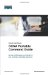# Binary ANDing

Binary ANDing is the process of performing multiplication to two binary numbers. In the Decimal Numbering system, ANDing is addition: 2 and 3 equals 5. In Decimal, there are an infinite number of answers when ANDing two numbers together. However, in the Binary Numbering system, the AND function yields only two possible outcomes, based on four different combinations. These outcomes, or answers, can be displayed in what is known as a truth table:

0 and 0 = 0
1 and 0 = 0
0 and 1 = 0
1 and 1 = 1

You use ANDing most often when comparing an IP address to its subnet mask. The end result of ANDing these two numbers together is to yield the network number of that address.

#### Question 1

 [click here] What is the network number of the IP address 192.168.100.115 if it has a subnet mask of 255.255.255.240?

#### Answer

A1:
 Step 1. Convert both the IP address and the subnet mask to binary: 192.168.100.115 = 11000000.10101000.01100100.01110011255.255.255.240 = 11111111.11111111.11111111.11110000 Step 2. Perform the AND operation to each pair of bits1 bit from the address ANDed to the corresponding bit in the subnet mask. Refer to the truth table for the possible outcomes: 192.168.100.115 = 11000000.10101000.01100100.01110011255.255.255.240 = 11111111.11111111.11111111.11110000ANDed result = 11000000.10101000.01100100.01110000 Step 3. Convert the answer back into decimal: 11000000.10101000.01100100.01110000 = 192.168.100.112 The IP address 192.168.100.115 belongs to the 192.168.100.112 network when a mask of 255.255.255.240 is used.

#### Question 2

 [click here] What is the network number of the IP address 192.168.100.115 if it has a subnet mask of 255.255.255.192? (Notice that the IP address is the same as in Question 1, but the subnet mask is different. What answer do you think you will get? The same one? Let's find out!)

#### Answer

A1:
 Step 1. Convert both the IP address and the subnet mask to binary: 192.168.100.115 = 11000000.10101000.01100100.01110011255.255.255.192 = 11111111.11111111.11111111.11000000 Step 2. Perform the AND operation to each pair of bits1 bit from the address ANDed to the corresponding bit in the subnet mask. Refer to the truth table for the possible outcomes: 192.168.100.115 = 11000000.10101000.01100100.01110011255.255.255.192 = 11111111.11111111.11111111.11000000ANDed result    = 11000000.10101000.01100100.01000000 Step 3. Convert the answer back into decimal: 11000000.10101000.01100100.01110000 = 192.168.100.64 The IP address 192.168.100.115 belongs to the 192.168.100.64 network when a mask of 255.255.255.192 is used.

#### So Why AND?

Good question. The best answer is to save you time when working with IP addressing and subnetting. If you are given an IP address and its subnet, you can quickly find out what subnetwork the address belongs to. From here, you can determine what OTHER addresses belong to the same subnet. Remember that if two addresses are in the same network or subnetwork, they are considered to be local to each other, and can therefore communicate directly with each other. Addresses that are not in the same network or subnetwork are considered to be remote to each other, and must therefore have a Layer 3 device (like a router or Layer 3 switch) between them in order to communicate.

##### Question 3

 [click here] What is the broadcast address of the IP address 192.168.100.164 if it has a subnet mask of 255.255.255.248?

##### Answer

A1:
 Step 1. Convert both the IP address and the subnet mask to binary: 192.168.100.164 = 11000000.10101000.01100100.10100100255.255.255.248 = 11111111.11111111.11111111.11111000 Step 2. Perform the AND operation to each pair of bits1 bit from the address ANDed to the corresponding bit in the subnet mask. Refer to the truth table for the possible outcomes: 192.168.100.164 = 11000000.10101000.01100100.10100100255.255.255.248 = 11111111.11111111.11111111.11111000ANDed Result    = 11000000.10101000.01100100.10100000                           = 192.168.100.160 (Subnetwork #) Step 3. Separate the network bits from the host bits: 255.255.255.248 = /29 = 1st 29 bits are network/subnetwork bits, therefore 11000000.10101000.01100100.10100000. The last three bits are host bits. Step 4. Change all host bits to 1. Remember that all 1s in the host portion are the broadcast number for that subnetwork: 11000000.10101000.01100100.10100111 Step 5. Convert this number to decimal to reveal your answer: 11000000.10101000.01100100.10100111 = 192.168.100.167 The broadcast address of 192.168.100.164 is 192.168.100.167 when the subnet mask is 255.255.255.248.

#### Shortcuts in Binary ANDing

Remember when I said that this was supposed to save you time when working with IP addressing and subnetting? Well, there are shortcuts when you AND two numbers together:

• An octet of all 1s in the subnet mask will result in the answer being the exact same octet as in the IP address

• An octet of all 0s in the subnet mask will result in the answer being all 0s in that octet

##### Question 4

 [click here] To what network does 172.16.100.45 belong, if its subnet mask is 255.255.255.0?

##### Answer

 A1: 172.16.100.0

##### Proof
 Step 1. Convert both the IP address and the subnet mask to binary: 172.16.100.45 = 10101100.00010000.01100100.00101101255.255.255.0 = 11111111.11111111.11111111.00000000 Step 2. Perform the AND operation to each pair of bits 1 bit from the address ANDed to the corresponding bit in the subnet mask. Refer to the truth table for the possible outcomes: 172.16.100.45 = 10101100.00010000.01100100.00101101255.255.255.0 = 11111111.11111111.11111111.00000000                           10101100.00010000.01100100.00000000                        = 172.16.100.0

Notice that the first three octets have the same pattern both before and after they were ANDed. Therefore, any octet ANDed to a subnet mask pattern of 255 is itself! Notice that the last octet is all 0s after ANDing. But according to the truth table, anything ANDed to a 0 is a 0. Therefore, any octet ANDed to a subnet mask pattern of 0 is 0! You should only have to convert those parts of an IP address and subnet mask to binary if the mask is not 255 or 0.

##### Question 5

 [click here] To what network does 68.43.100.18 belong, if its subnet mask is 255.255.255.0?

##### Answer

 A1: 68.43.100.0 (No need to convert here, mask is either 255s or 0s.)

##### Question 6

 [click here] To what network does 131.186.227.43 belong, if its subnet mask is 255.255.240.0?

##### Answer

 A1: Based on the two shortcut rules, the answer should be 131.186.???.0 So now you only need to convert one octet to binary for the ANDing process: 227 =      11100011240 =      1111000011100000 = 224 Therefore the answer is 131.186.224.0.CCNA Portable Command Guide
ISBN: 1587201585
EAN: 2147483647
Year: 2006
Pages: 261
Authors: Scott Empson

Similar book on Amazon

flylib.com © 2008-2017.
If you may any questions please contact us: flylib@qtcs.net